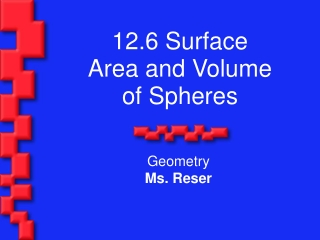DownloadDownload Presentation12.6 Surface Area and Volume of Spheres

# 12.6 Surface Area and Volume of Spheres

Télécharger la présentation## 12.6 Surface Area and Volume of Spheres

- - - - - - - - - - - - - - - - - - - - - - - - - - - E N D - - - - - - - - - - - - - - - - - - - - - - - - - - -
##### Presentation Transcript

1. 12.6 Surface Area and Volume of Spheres Geometry Ms. Reser

2. Objectives/Assignment • Find the surface area of a sphere. • Find the volume of a sphere in real life such as the ball bearing in Ex. 4. • 12.6 WS A

3. Finding the Surface Area of a Sphere • In Lesson 10.7, a circle was described as a locus of points in a plane that are a given distance from a point. A sphere is the locus of points in space that are a given distance from a point.

4. Finding the Surface Area of a Sphere • The point is called the center of the sphere. A radius of a sphere is a segment from the center to a point on the sphere. • A chord of a sphere is a segment whose endpoints are on the sphere.

5. Finding the Surface Area of a Sphere • A diameter is a chord that contains the center. As with all circles, the terms radius and diameter also represent distances, and the diameter is twice the radius.

6. Theorem 12.11: Surface Area of a Sphere • The surface area of a sphere with radius r is S = 4r2.

7. Ex. 1: Finding the Surface Area of a Sphere • Find the surface area. When the radius doubles, does the surface area double?

8. S = 4r2 = 422 = 16 in.2 S = 4r2 = 442 = 64 in.2 The surface area of the sphere in part (b) is four times greater than the surface area of the sphere in part (a) because 16 • 4 = 64 So, when the radius of a sphere doubles, the surface area DOES NOT double.

9. More . . . • If a plane intersects a sphere, the intersection is either a single point or a circle. If the plane contains the center of the sphere, then the intersection is a great circle of the sphere. Every great circle of a sphere separates a sphere into two congruent halves called hemispheres.

10. Ex. 2: Using a Great Circle • The circumference of a great circle of a sphere is 13.8 feet. What is the surface area of the sphere?

11. Solution: Begin by finding the radius of the sphere. C = 2r 13.8 = 2r 13.8 2r 6.9 = r = r

12. Solution: Using a radius of 6.9 feet, the surface area is: S = 4r2 = 4(6.9)2 = 190.44 ft.2 So, the surface area of the sphere is 190.44  ft.2

13. Ex. 3: Finding the Surface Area of a Sphere • Baseball. A baseball and its leather covering are shown. The baseball has a radius of about 1.45 inches. • Estimate the amount of leather used to cover the baseball. • The surface area of a baseball is sewn from two congruent shapes, each which resembles two joined circles. How does this relate to the formula for the surface area of a sphere?

14. Ex. 3: Finding the Surface Area of a Sphere

15. Finding the Volume of a Sphere • Imagine that the interior of a sphere with radius r is approximated by n pyramids as shown, each with a base area of B and a height of r, as shown. The volume of each pyramid is 1/3 Br and the sum is nB.

16. Finding the Volume of a Sphere • The surface area of the sphere is approximately equal to nB, or 4r2. So, you can approximate the volume V of the sphere as follows:

17. V  n(1/3)Br = 1/3 (nB)r  1/3(4r2)r =4/3r2 Each pyramid has a volume of 1/3Br. Regroup factors. Substitute 4r2 for nB. Simplify. More . . .

18. Theorem 12.12: Volume of a Sphere • The volume of a sphere with radius r is S = 4r3. 3

19. Ex. 4: Finding the Volume of a Sphere • Ball Bearings. To make a steel ball bearing, a cylindrical slug is heated and pressed into a spherical shape with the same volume. Find the radius of the ball bearing to the right:

20. Solution: • To find the volume of the slug, use the formula for the volume of a cylinder. V = r2h = (12)(2) = 2 cm3 To find the radius of the ball bearing, use the formula for the volume of a sphere and solve for r.

21. V =4/3r3 2 = 4/3r3 6 = 4r3 1.5 = r3 1.14  r Formula for volume of a sphere. Substitute 2 for V. Multiply each side by 3. Divide each side by 4. Use a calculator to take the cube root. More . . . So, the radius of the ball bearing is about 1.14 cm.